#### Spanning tree covering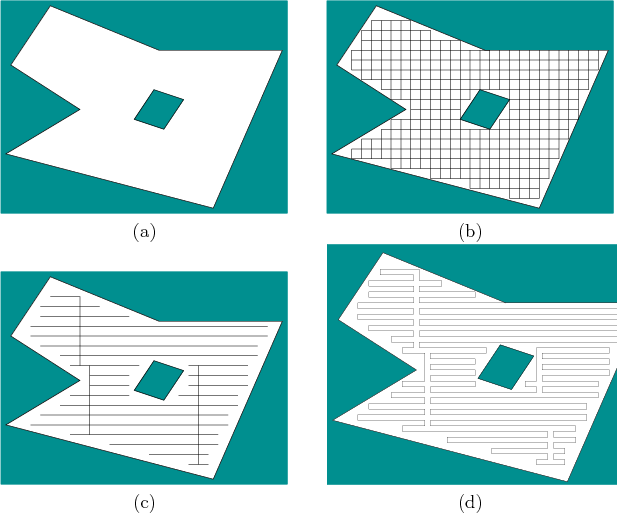An interesting approximate method was developed by Gabriely and Rimon; it places a tiling of squares inside ofand computes the spanning tree of the resulting connectivity graph [372,373]. Suppose again thatis polygonal. Consider the example shown in Figure 7.37a. The first step is to tile the interior ofwith squares, as shown in Figure 7.37b. Each square should be of width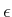, for some constant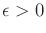. Next, construct a roadmap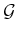by placing a vertex in the center of each square and by defining an edge that connects the centers of each pair of adjacent cubes. The next step is to compute a spanning tree of. This is a connected subgraph that has no cycles and touches every vertex of; it can be computed in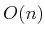time, ifhasedges . There are many possible spanning trees, and a criterion can be defined and optimized to induce preferences. One possible spanning tree is shown Figure 7.37c.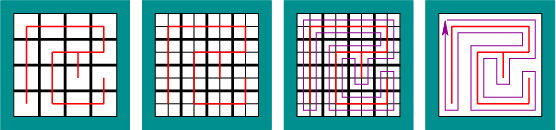Once the spanning tree is made, the robot path is obtained by starting at a point near the spanning tree and following along its perimeter. This path can be precisely specified as shown in Figure 7.38. Double the resolution of the tiling, and form the corresponding roadmap. Part of the roadmap corresponds to the spanning tree, but also included is a loop path that surrounds the spanning tree. This path visits the centers of the new squares. The resulting path for the example of Figure 7.37a is shown in Figure 7.37d. In general, the method yields an optimal route, once the approximation is given. A bound on the uncovered area due to approximation is given in . Versions of the method that do not require an initial map are also given in [372,373]; this involves reasoning about information spaces, which are covered in Chapter 11.

Steven M LaValle 2020-08-14